Скачать презентацию MA 3264 Mathematical Modeling Weizhu Bao Department of

1f63599b80dc447ba5d8c6467e078d16.ppt

• Количество слайдов: 24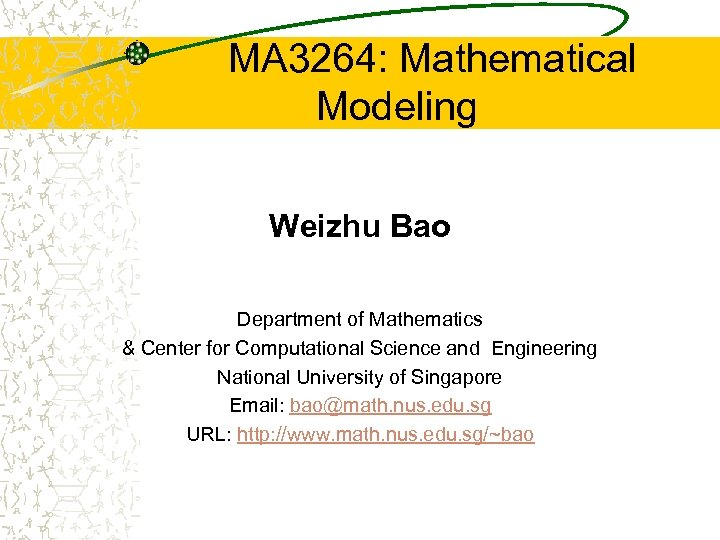MA 3264: Mathematical Modeling Weizhu Bao Department of Mathematics & Center for Computational Science and Engineering National University of Singapore Email: [email protected] nus. edu. sg URL: http: //www. math. nus. edu. sg/~bao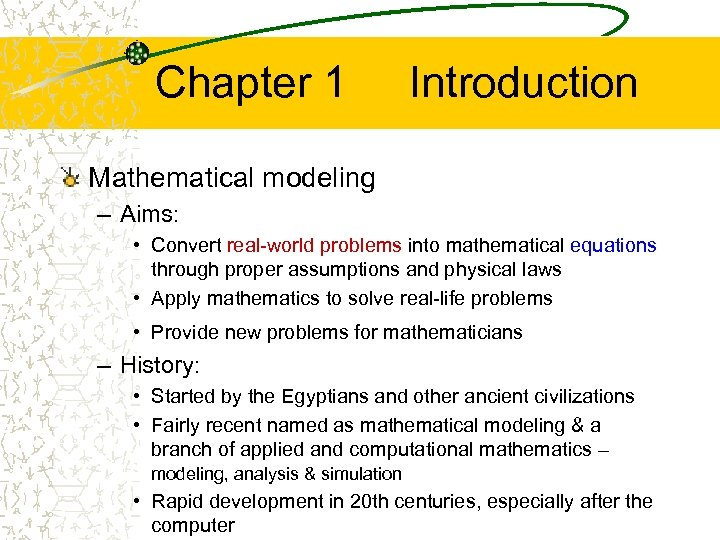Chapter 1 Introduction Mathematical modeling – Aims: • Convert real-world problems into mathematical equations through proper assumptions and physical laws • Apply mathematics to solve real-life problems • Provide new problems for mathematicians – History: • Started by the Egyptians and other ancient civilizations • Fairly recent named as mathematical modeling & a branch of applied and computational mathematics – modeling, analysis & simulation • Rapid development in 20 th centuries, especially after the computer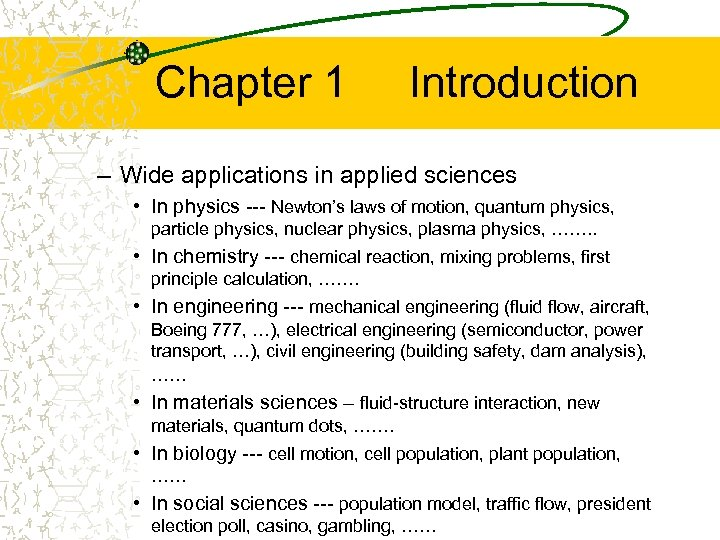Chapter 1 Introduction – Wide applications in applied sciences • In physics --- Newton’s laws of motion, quantum physics, particle physics, nuclear physics, plasma physics, ……. . • In chemistry --- chemical reaction, mixing problems, first principle calculation, ……. • In engineering --- mechanical engineering (fluid flow, aircraft, Boeing 777, …), electrical engineering (semiconductor, power transport, …), civil engineering (building safety, dam analysis), …… • In materials sciences – fluid-structure interaction, new materials, quantum dots, ……. • In biology --- cell motion, cell population, plant population, …… • In social sciences --- population model, traffic flow, president election poll, casino, gambling, ……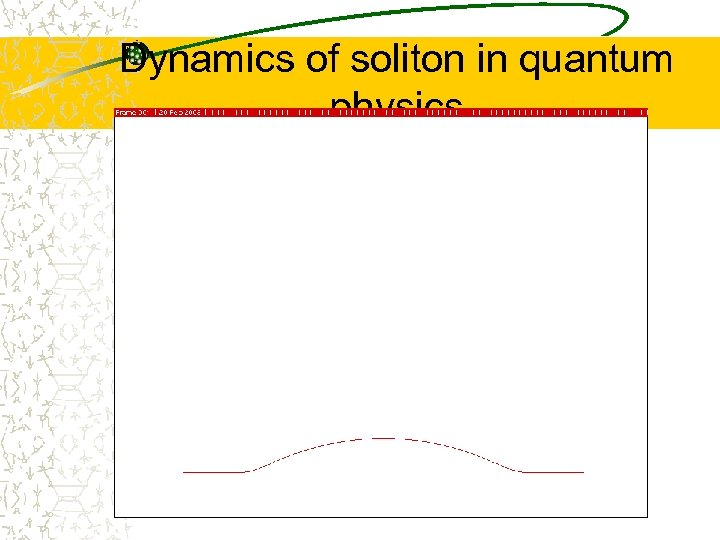Dynamics of soliton in quantum physics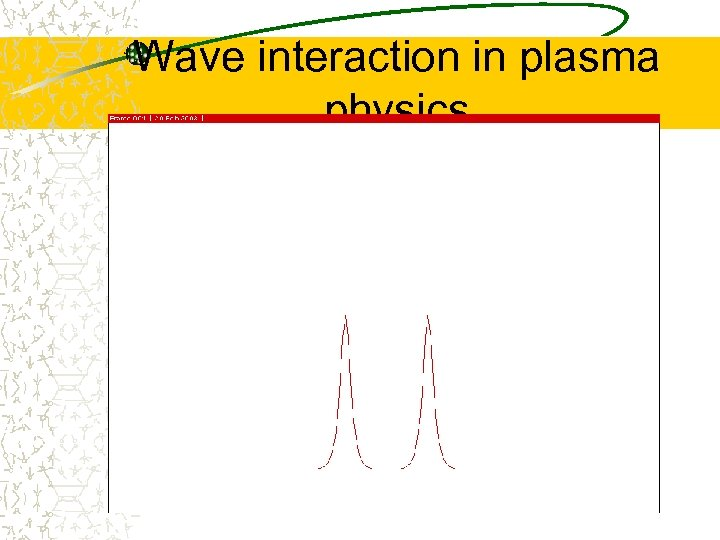Wave interaction in plasma physics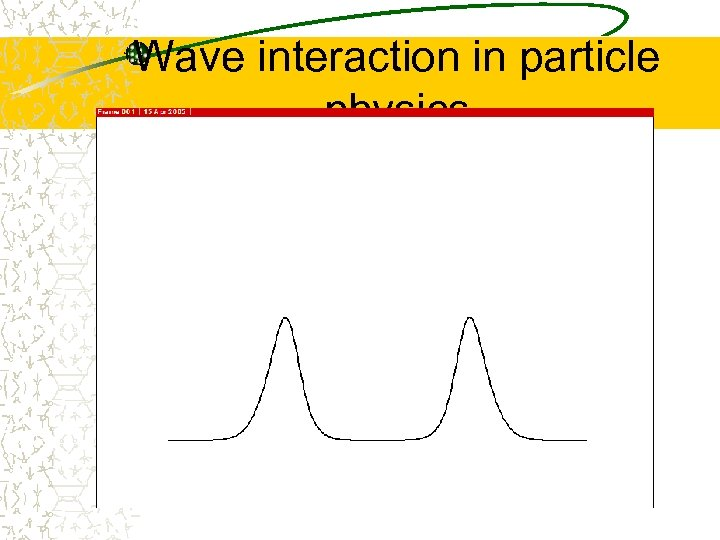Wave interaction in particle physics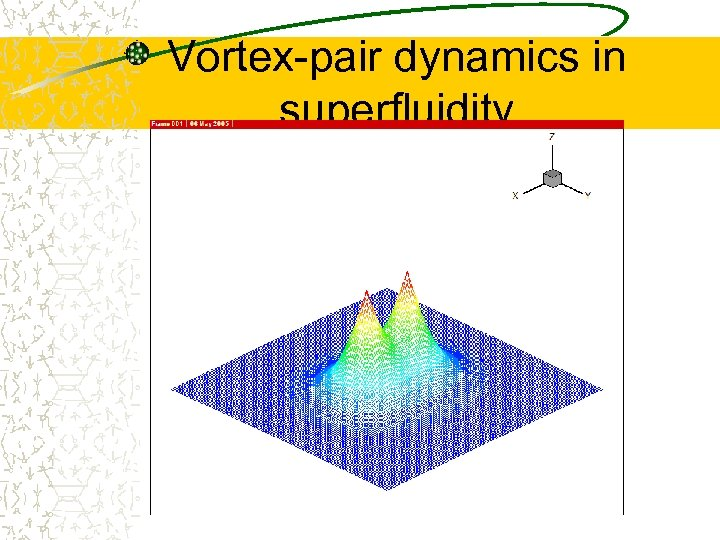Vortex-pair dynamics in superfluidity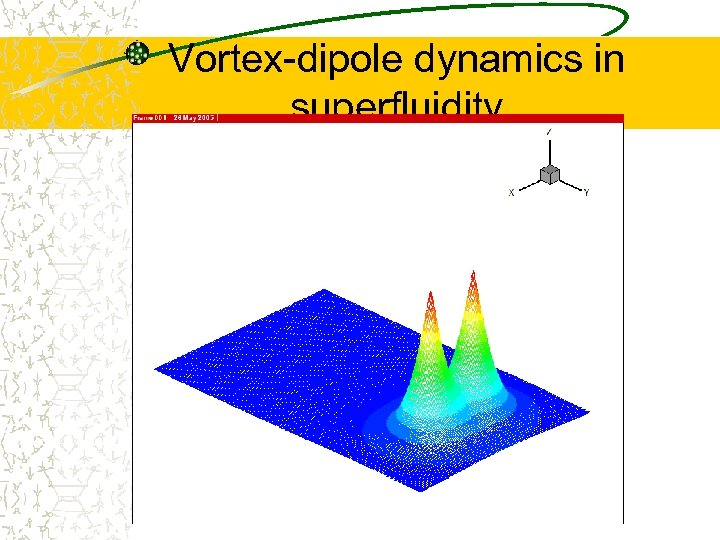Vortex-dipole dynamics in superfluidity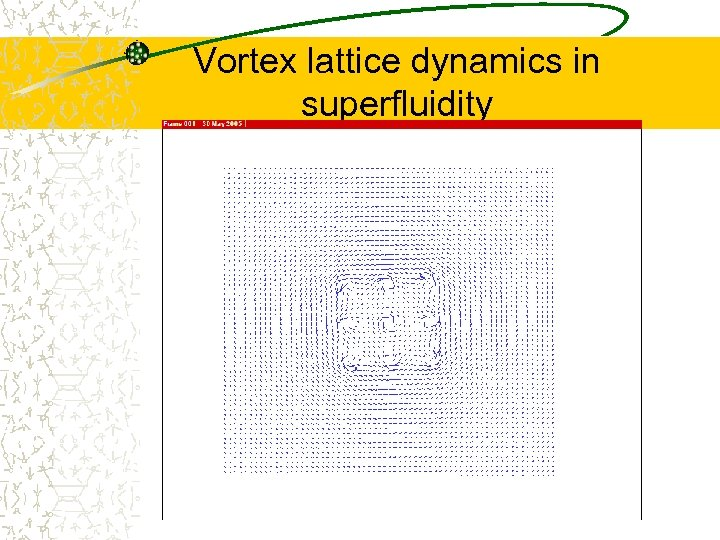Vortex lattice dynamics in superfluidity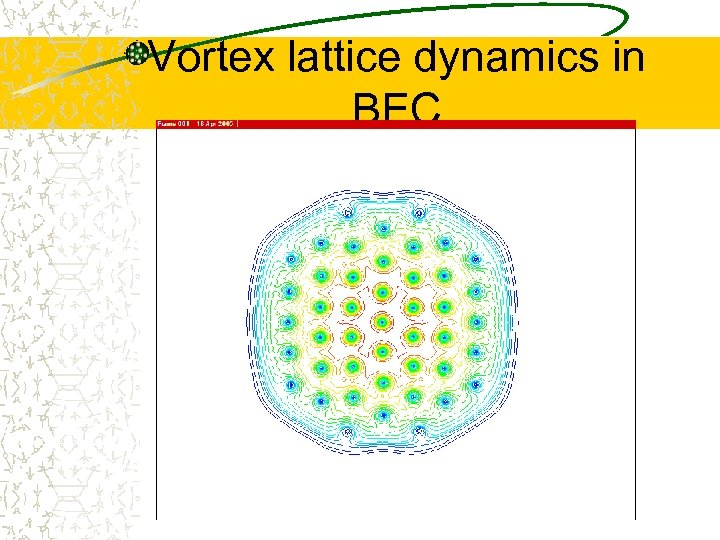Vortex lattice dynamics in BEC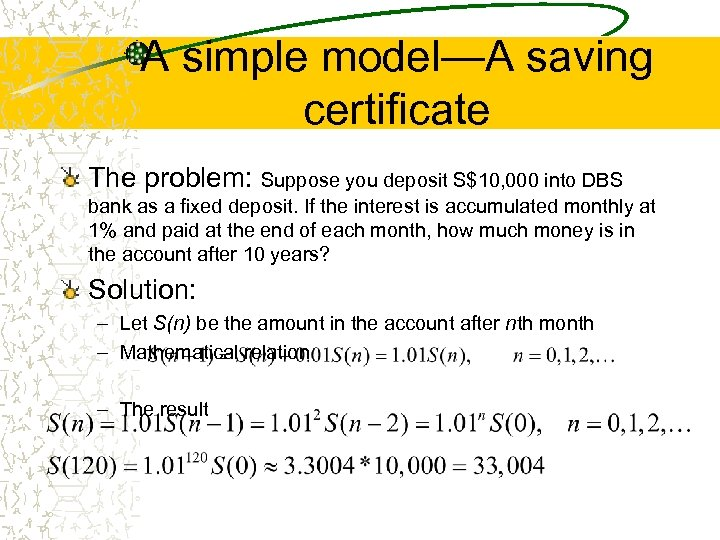A simple model—A saving certificate The problem: Suppose you deposit S\$10, 000 into DBS bank as a fixed deposit. If the interest is accumulated monthly at 1% and paid at the end of each month, how much money is in the account after 10 years? Solution: – Let S(n) be the amount in the account after nth month – Mathematical relation – The result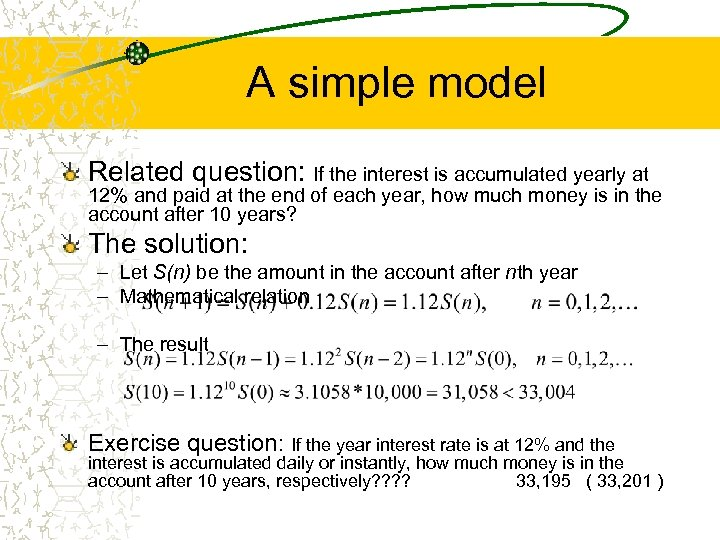A simple model Related question: If the interest is accumulated yearly at 12% and paid at the end of each year, how much money is in the account after 10 years? The solution: – Let S(n) be the amount in the account after nth year – Mathematical relation – The result Exercise question: If the year interest rate is at 12% and the interest is accumulated daily or instantly, how much money is in the account after 10 years, respectively? ? 33, 195 ( 33, 201 )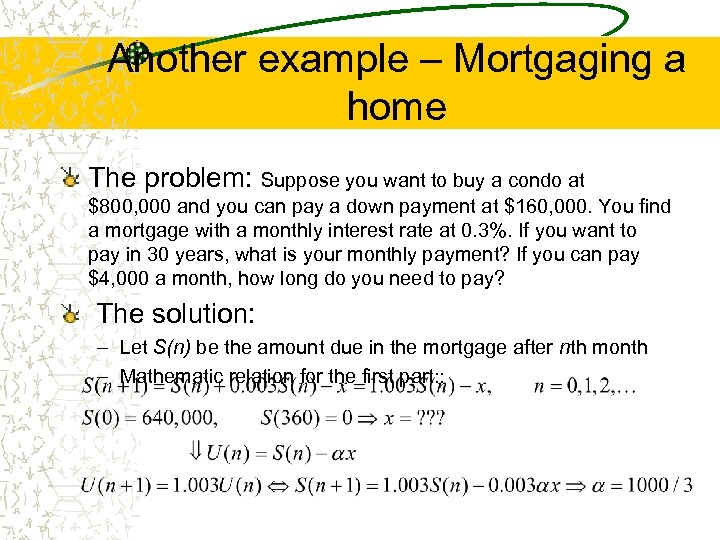Another example – Mortgaging a home The problem: Suppose you want to buy a condo at \$800, 000 and you can pay a down payment at \$160, 000. You find a mortgage with a monthly interest rate at 0. 3%. If you want to pay in 30 years, what is your monthly payment? If you can pay \$4, 000 a month, how long do you need to pay? The solution: – Let S(n) be the amount due in the mortgage after nth month – Mathematic relation for the first part: :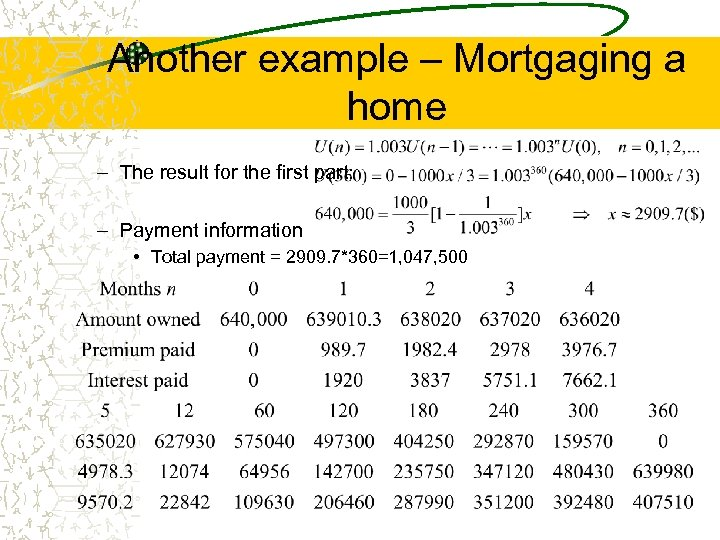Another example – Mortgaging a home – The result for the first part: – Payment information • Total payment = 2909. 7*360=1, 047, 500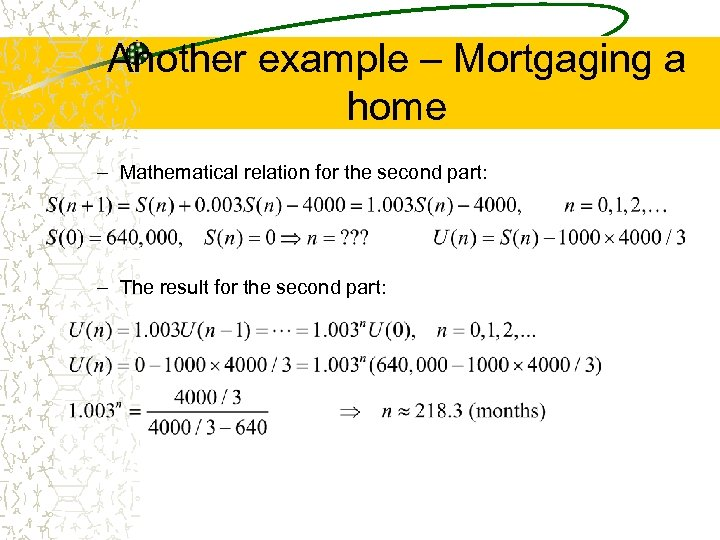Another example – Mortgaging a home – Mathematical relation for the second part: – The result for the second part: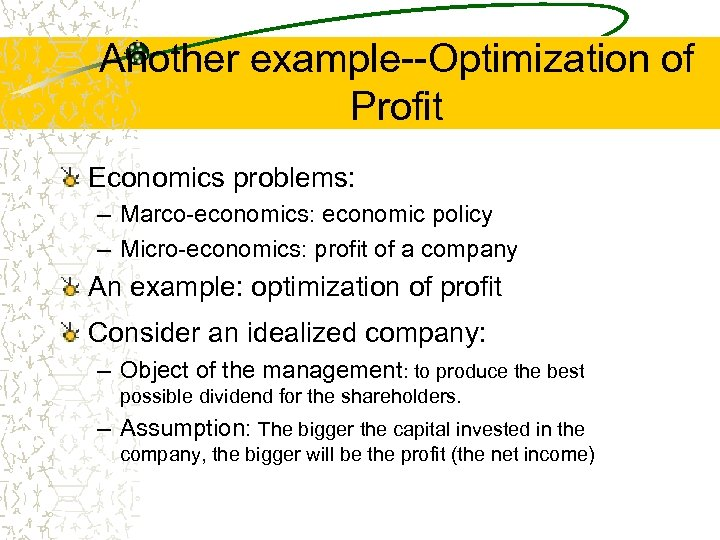Another example--Optimization of Profit Economics problems: – Marco-economics: economic policy – Micro-economics: profit of a company An example: optimization of profit Consider an idealized company: – Object of the management: to produce the best possible dividend for the shareholders. – Assumption: The bigger the capital invested in the company, the bigger will be the profit (the net income)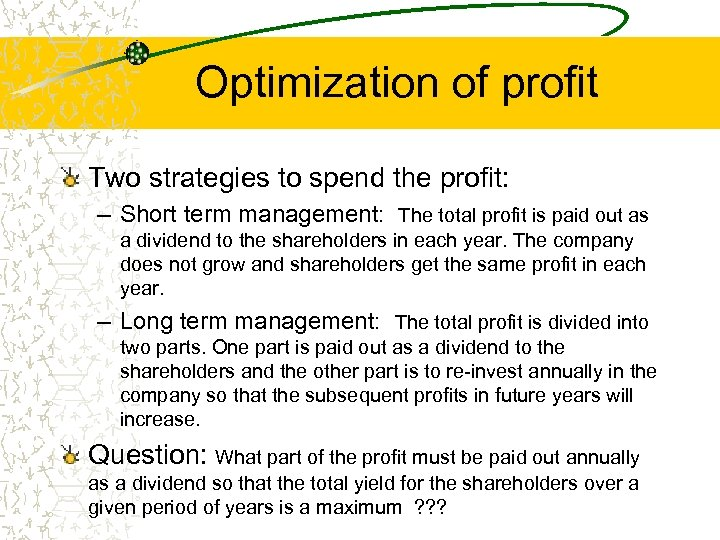Optimization of profit Two strategies to spend the profit: – Short term management: The total profit is paid out as a dividend to the shareholders in each year. The company does not grow and shareholders get the same profit in each year. – Long term management: The total profit is divided into two parts. One part is paid out as a dividend to the shareholders and the other part is to re-invest annually in the company so that the subsequent profits in future years will increase. Question: What part of the profit must be paid out annually as a dividend so that the total yield for the shareholders over a given period of years is a maximum ? ? ?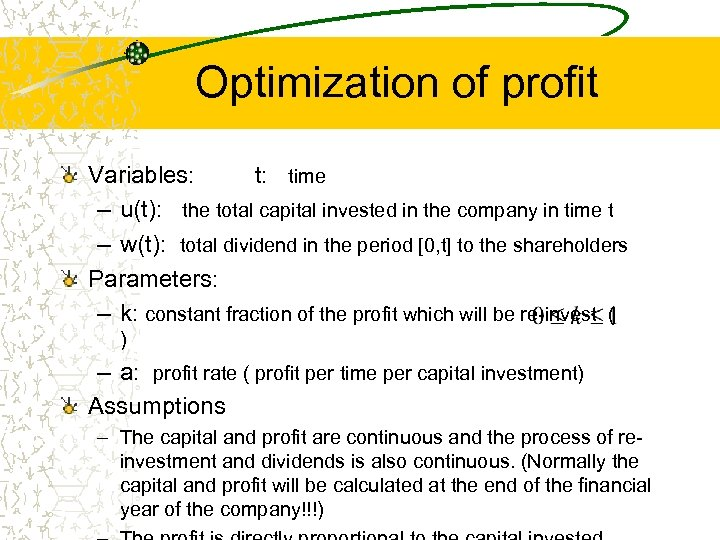Optimization of profit Variables: t: time – u(t): the total capital invested in the company in time t – w(t): total dividend in the period [0, t] to the shareholders Parameters: – k: constant fraction of the profit which will be re-invest ( ) – a: profit rate ( profit per time per capital investment) Assumptions – The capital and profit are continuous and the process of reinvestment and dividends is also continuous. (Normally the capital and profit will be calculated at the end of the financial year of the company!!!)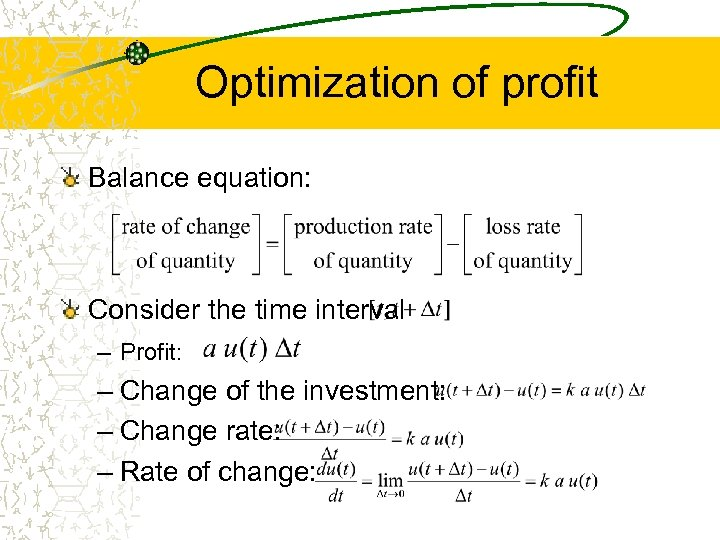Optimization of profit Balance equation: Consider the time interval – Profit: – Change of the investment: – Change rate: – Rate of change: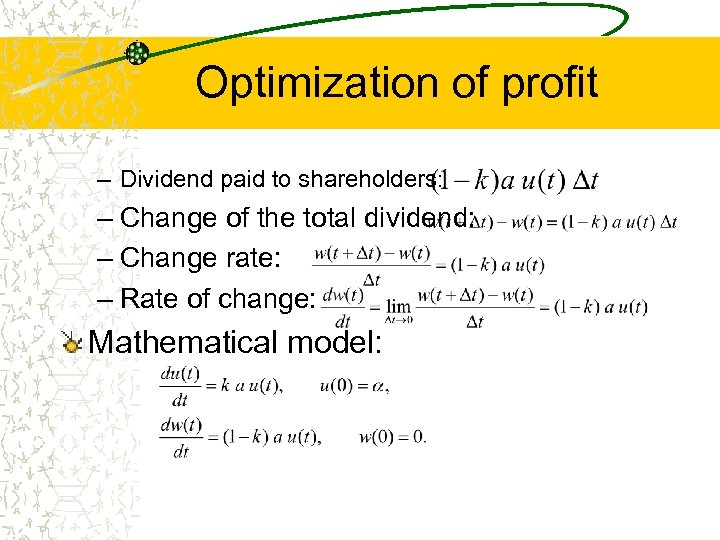Optimization of profit – Dividend paid to shareholders: – Change of the total dividend: – Change rate: – Rate of change: Mathematical model: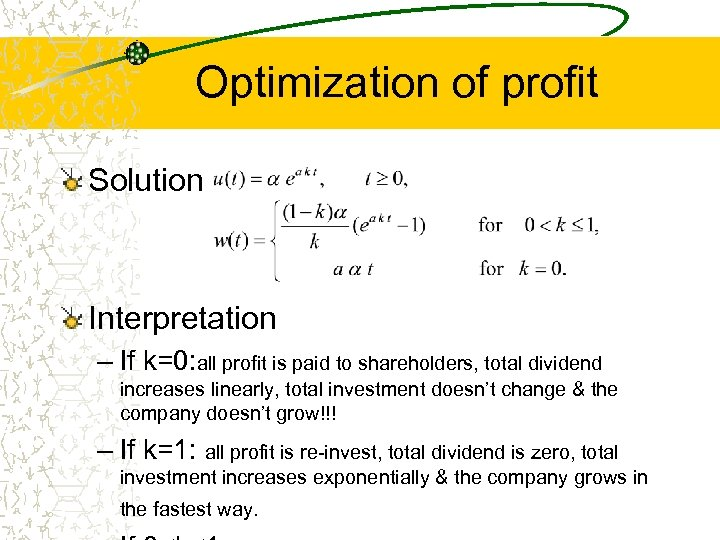Optimization of profit Solution Interpretation – If k=0: all profit is paid to shareholders, total dividend increases linearly, total investment doesn’t change & the company doesn’t grow!!! – If k=1: all profit is re-invest, total dividend is zero, total investment increases exponentially & the company grows in the fastest way.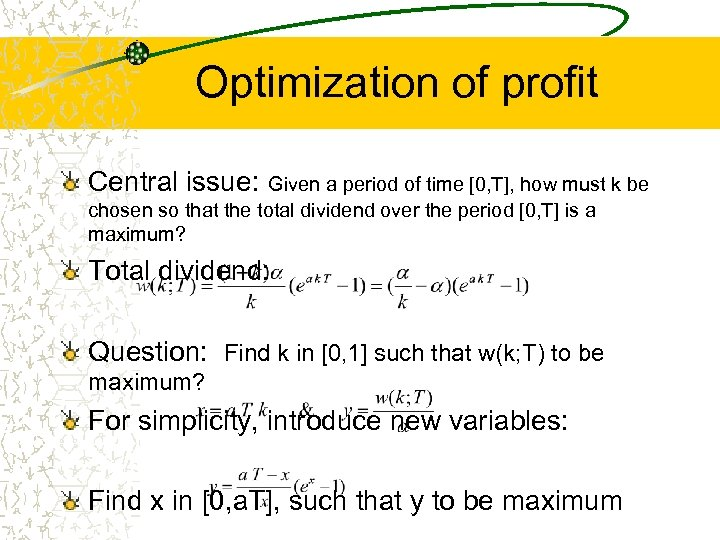Optimization of profit Central issue: Given a period of time [0, T], how must k be chosen so that the total dividend over the period [0, T] is a maximum? Total dividend: Question: Find k in [0, 1] such that w(k; T) to be maximum? For simplicity, introduce new variables: Find x in [0, a. T], such that y to be maximum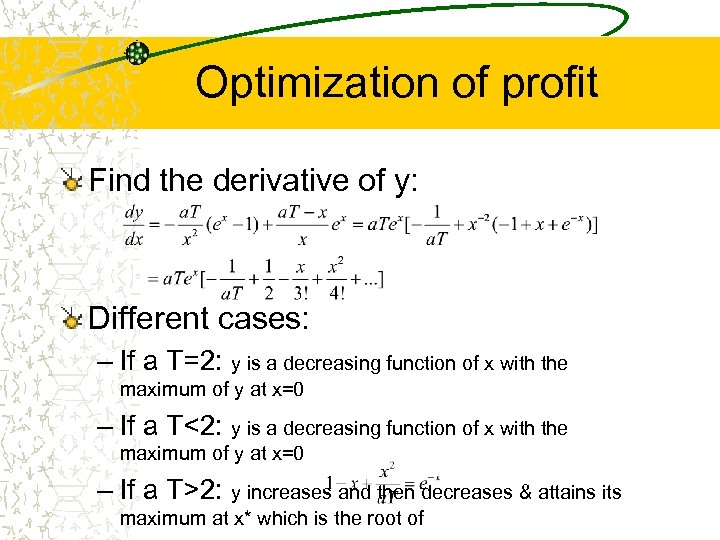Optimization of profit Find the derivative of y: Different cases: – If a T=2: y is a decreasing function of x with the maximum of y at x=0 – If a T<2: y is a decreasing function of x with the maximum of y at x=0 – If a T>2: y increases and then decreases & attains its maximum at x* which is the root of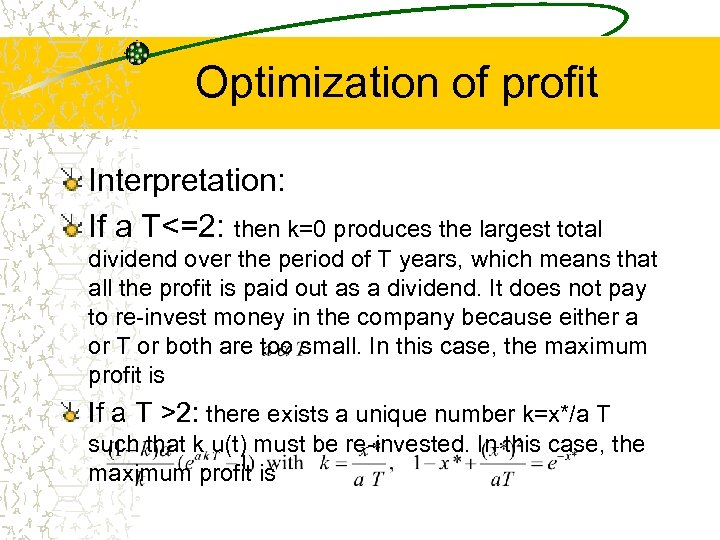Optimization of profit Interpretation: If a T<=2: then k=0 produces the largest total dividend over the period of T years, which means that all the profit is paid out as a dividend. It does not pay to re-invest money in the company because either a or T or both are too small. In this case, the maximum profit is If a T >2: there exists a unique number k=x*/a T such that k u(t) must be re-invested. In this case, the maximum profit is